Special Issue in honor of Alan C. Lazer. Electron. J. Diff. Eqns., Special Issue 01 (2021), pp. 23-89.

### Space-time analyticity of weak solutions to semilinear parabolic systems with variable coefficients Falko Baustian, Peter Takac

Abstract:
We study analytic smooth solutions of a general, strongly parabolic semilinear Cauchy problem of 2m-th order in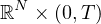with analytic coefficients (in space and time variables) and analytic initial data (in space variables). They are expressed in terms of holomorphic continuation of global (weak) solutions to the system valued in a suitable Besov interpolation space of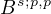-type at every time moment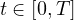. Given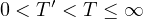, it is proved that any-type solution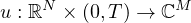with analytic initial data possesses a bounded holomorphic continuation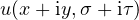into a complex domain in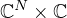defined by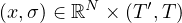,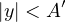and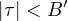, where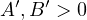are constants depending upon T'. The proof uses the extension of a weak solution to a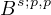-type solution in a domain in, such that this extension satisfies the Cauchy-Riemann equations. The holomorphic extension is obtained with a help from holomorphic semigroups and maximal regularity theory for parabolic problems in Besov interpolation spaces of-type imbedded (densely and continuously) into an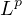-type Lebesgue space. Applications include risk models for European options in Mathematical Finance.

Published October 6, 2021.
Math Subject Classifications: 35B65, 35K10, 32D05, 91G40.
Key Words: Space-time analyticity; parabolic PDE; holomorphic semigroup; Besov space; maximal regularity; Hardy space; holomorphic continuation to a complex strip; European option; bilateral counterparty risk.

Show me the PDF file (795 K), TEX file for this article.Falko Baustian Institut für Mathematik Universität Rostock Ulmenstraße 69, Haus 3 D-18051 Rostock, Germany email: falko.baustian@uni-rostock.de Peter Takác Institut für Mathematik Universität Rostock Ulmenstraße 69, Haus 3 D-18051 Rostock, Germany https://www.mathematik.uni-rostock.de/struktur/lehrstuehle/angewandte-analysis email: peter.takac@uni-rostock.de